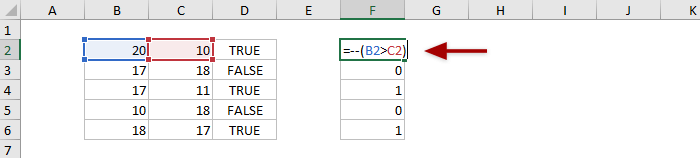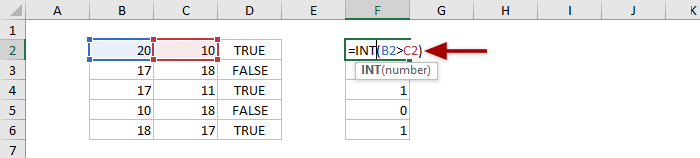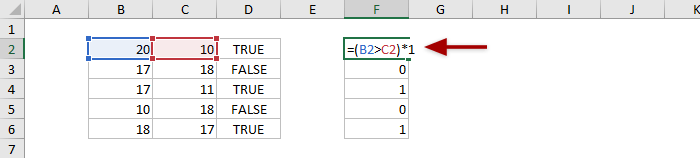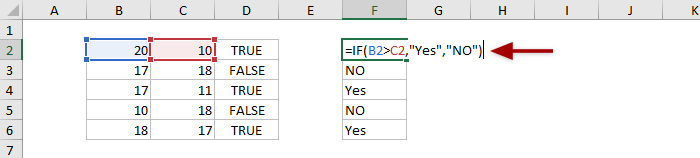## How to convert Boolean true/false to number (1/0) or text in Excel?

Sometimes, excel formulas return the Boolean values (TRUE or FALSE) in Excel. And you can edit the original formula to convert the Boolean values (TRUE or FALSE) to number (1 or 0) or text in Excel easily.

#### Convert Boolean values (TRUE or FALSE) to number (1 or 0) in Excel

In this section, I will introduce three methods to convert Boolean values (TRUE or FALSE) to number (1 or 0) in Excel.

##### Add -- before original formula

Change original formula to =--(original_formula), and the Boolean values will be converted to number 1 or 0 automatically. For example, the original formula is =B2>C2, you can change it to =--(B2>C2).##### Combine INT function and original formula

Combine the INT function and original formula as =INT(original_formula), and the Boolean values will be converted to number 1 or 0 automatically. Let’s say the original formula is =B2>C2, and you can convert it to =INT(B2>C2).##### Multiply original formula by 1

You can multiply the return Boolean values (TRUE or FALSE) by 1, and then the TRUE will change to 1, and FALSE to 0. Assuming the original formula is =B2>C2, you can change it to =(B2>C2)*1.Note: You can also divide original formula by 1 or add 0 to original formula to change the return TRUE to 1 and FALSE to 0. Follow above example, change the original formula to =(B2>C2)/1 or =(B2>C2)+0.

#### Convert Boolean values (TRUE or FALSE) to text in Excel

If you want to convert the Boolean values (TRUE or FALSE) to certain text, says Yes or No, you can change the formula as below:

=IF(original_formula,"Yes","NO")

For example, the original formula is =B2>C2, you can change the formula to =IF(B2>C2,"Yes","NO"). This new formula will change TRUE to Yes, and change FALSE to No. See screenshot:Note: In the new formula, Yes and No are the specified texts you will change the Boolean values to, and you can change them to any texts as you need.

### Best Office Productivity Tools

 Popular Features: Find, Highlight or Identify Duplicates   |  Delete Blank Rows   |  Combine Columns or Cells without Losing Data   |   Round without Formula ... Super Lookup: Multiple Criteria VLookup  |   Multiple Value VLookup  |   VLookup Across Multiple Sheets   |   Fuzzy Lookup .... Advanced Drop-down List: Quickly Create Drop Down List   |  Dependent Drop Down List   |  Multi-select Drop Down List .... Column Manager: Add a Specific Number of Columns  |  Move Columns  |  Toggle Visibility Status of Hidden Columns  |  Compare Ranges & Columns ... Featured Features: Grid Focus   |  Design View   |   Big Formula Bar   |  Workbook & Sheet Manager   |  Resource Library (Auto Text)   |  Date Picker   |  Combine Worksheets   |  Encrypt/Decrypt Cells   |  Send Emails by List   |  Super Filter   |   Special Filter (filter bold/italic/strikethrough...) ... Top 15 Toolsets:  12 Text Tools (Add Text, Remove Characters, ...)   |   50+ Chart Types (Gantt Chart, ...)   |   40+ Practical Formulas (Calculate age based on birthday, ...)   |   19 Insertion Tools (Insert QR Code, Insert Picture from Path, ...)   |   12 Conversion Tools (Numbers to Words, Currency Conversion, ...)   |   7 Merge & Split Tools (Advanced Combine Rows, Split Cells, ...)   |   ... and more

Supercharge Your Excel Skills with Kutools for Excel, and Experience Efficiency Like Never Before. Kutools for Excel Offers Over 300 Advanced Features to Boost Productivity and Save Time.  Click Here to Get The Feature You Need The Most...#### Office Tab Brings Tabbed interface to Office, and Make Your Work Much Easier

• Enable tabbed editing and reading in Word, Excel, PowerPoint, Publisher, Access, Visio and Project.
• Open and create multiple documents in new tabs of the same window, rather than in new windows.
• Increases your productivity by 50%, and reduces hundreds of mouse clicks for you every day!